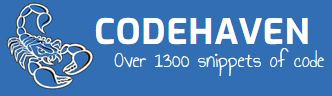Generic filters
Exact matches only

# Current time/date (2.7)

19th November 2014

## Python%a Weekday name.
%A Full weekday name.
%b Abbreviated month name.
%B Full month name.
%c Appropriate date and time representation.
%d Day of the month as a decimal number [01,31].
%H Hour (24-hour clock) as a decimal number [00,23].
%I Hour (12-hour clock) as a decimal number [01,12].
%j Day of the year as a decimal number [001,366].
%m Month as a decimal number [01,12].
%M Minute as a decimal number [00,59].
%p Equivalent of either AM or PM.
%S Second as a decimal number [00,61].
%U Week number of the year (Sunday as the first day of the week) as a decimal number [00,53]. All days in a new year preceding the first Sunday are considered to be in week 0.
%w Weekday as a decimal number [0(Sunday),6].
%W Week number of the year (Monday as the first day of the week) as a decimal number [00,53]. All days in a new year preceding the first Monday are considered to be in week 0.
%x Appropriate date representation.
%X Apropriate time representation.
%y Year without century as a decimal number [00,99].
%Y Year with century as a decimal number.
%Z Time zone name (no characters if no time zone exists).
%% A literal ‘%’ character.